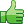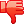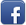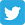# Pagination

Posted on 16th Feb 2014 07:03 pm by admin

Hi All,

I think I'm finally getting somewhere with pagination!

I can now submit a query and get the correct number of records back with the correct number of pages.

My only problem is, when I press the page numbers or the next button the records disappear.

If anyone gets time, could you take a look at my code and tell me where Iâ€™m going wrong please?

Jamie

Code: [Select]<?php

require("conf.php");

//max displayed per page
\$per_page = 2;

//get start variable
\$start = \$_GET['start'];

\$udfin = \$_POST['udf'];

//count records
\$record_count = mysql_num_rows(mysql_query("SELECT * FROM cy_equ WHERE udf = \$udfin"));

//count max pages
\$max_pages = \$record_count / \$per_page; //may come out as decimal

if (!\$start)
\$start = 0;

//display data
\$get = mysql_query("SELECT * FROM cy_equ WHERE udf = \$udfin LIMIT \$start, \$per_page");
while (\$row = mysql_fetch_assoc(\$get))
{
// get data
\$id = \$row['id'];
\$ext = \$row['ext'];
\$equ = \$row['equ'];

echo \$id." (".\$ext.") (".\$equ.")<br />";

}

//setup prev and next variables
\$prev = \$start - \$per_page;
\$next = \$start + \$per_page;

//show prev button
if (!(\$start<=0))
echo "<a href='qqq.php?start=\$prev'>Prev</a> ";

//show page numbers

//set variable for first page
\$i=1;

for (\$x=0;\$x<\$record_count;\$x=\$x+\$per_page)
{
if (\$start!=\$x)
echo " <a href='qqq.php?start=\$x'>\$i</a> ";
else
echo " <a href='qqq.php?start=\$x'><b>\$i</b></a> ";
\$i++;
}

//show next button
if (!(\$start>=\$record_count-\$per_page))
echo " <a href='qqq.php?start=\$next'>Next</a>";

?>
<h3> UDF Query </h3>
<form name="form1" method="post" action="http://localhost/qqq.php">
<p> Input UDF: <input type="number" name="udf" value= "<?php echo \$udfin; ?>" size="1" maxlength="4"/></p>

<p><input type="submit" name="submit" value="GET DATA"/></p>

</form>

5536##### Other forums

How to ask a regex question?
Hi, I thought I'd share some of my insight after seeing too many bad questions that either get ignor

Dear All,

I implemented Note OSS 1422843.

When i execute the new report RSPO20

I have an upload script that may be accessed from different directories. Problem is, the script is m

ob_ dynamic content
i was wondering about the potential to use ob to create a <div then remove it when the page

xml element exists
Code: [Select]<?xml version="1.0"?>
<

I am having issues getting a page to reload after I submit data to a database. This form should inp

DateObject and Nulls
Hi all,

I have an array mapped to a value object. One of the items in the array is a PHP Date

How to display random record from table?
I have the following code:

Code: <?php
\$display_block .= "<input type=

ALV List Display to point to another report on Double Click
Hi,

I want my ALV List Display to point to another report on Double Click on its line ite

Calling All PHPFREAKS Blackberry Users
Are you a member of PHPFreaks and have a blackberry? Well RIM just recently released Blackberry Mes